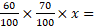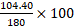General Mathematics Paper 2, WASSCE (PC), 2018

Question 7

1. A shop had two reduction sales during which prices of all items were reduced by 40% in the first sales and 30% in the second.

1. If a shirt was sold at GH₵35.00 during the second reduction sales, find the price before the first sale.
2. If the price of an article before the first reduction was GH₵180.00, find the total:
3. reduction in the price due to the two sales;
4. percentage reduction in the price of the article.

Observation

The Chief Examiner reported that only a negligible percentage of the Candidates’ applied the correct percentage at appropriate stage.
In part (a), let the original price of the shirt be x, then we have two percentages reduction leaving 60% and 70%. This implies that35.

Solving for x yields x = GH₵ 83.33.
In part (b) (i), the first reduction = (40/100) x 180 = 72.00 and the second reduction = (30 / 100) x 108 = 32.40. Then total reduction = (72.00 + 32. 40) = GH₵ 104.40.
In part (b) (ii), the percentage reduction in the price of the article == 58%.